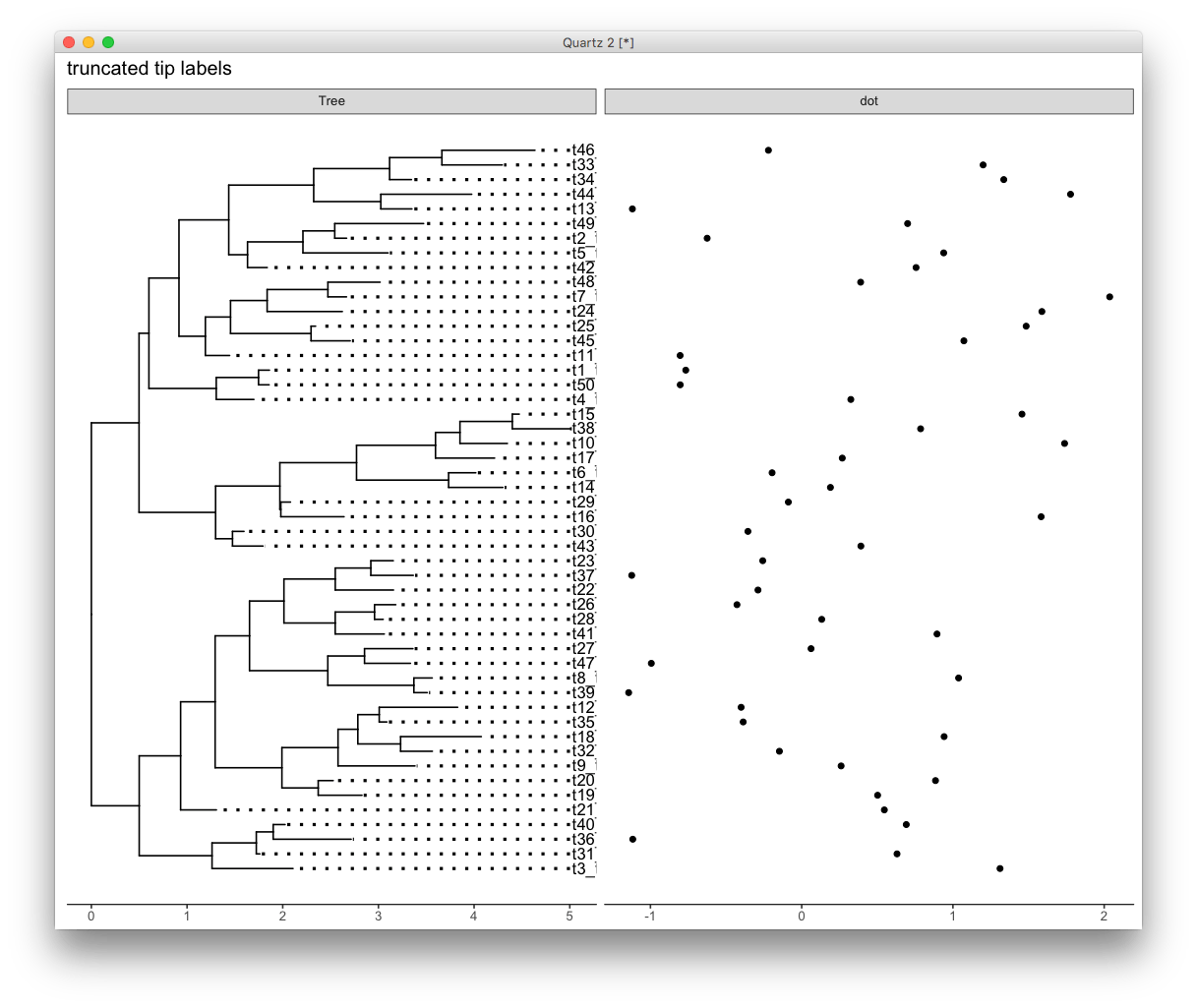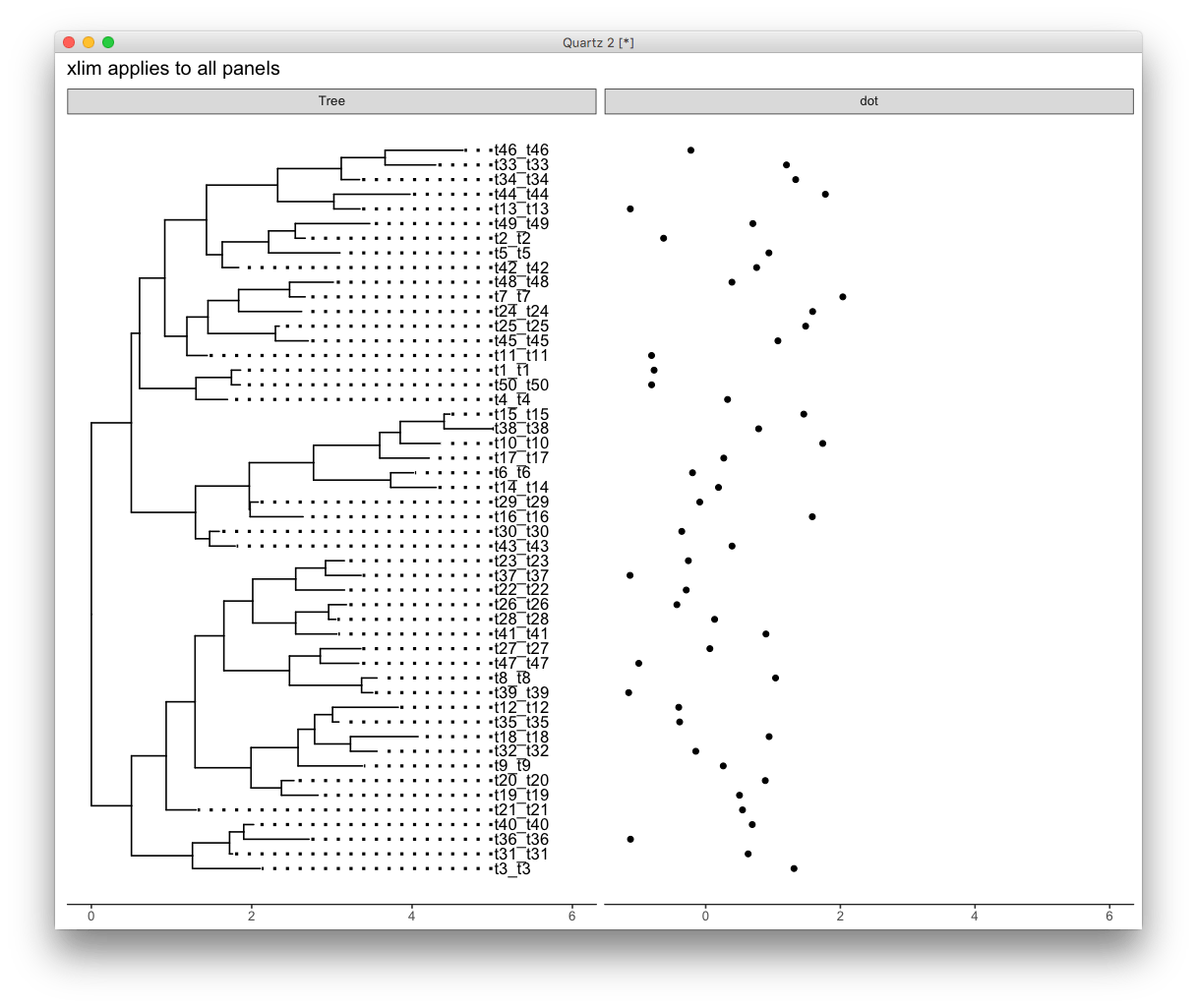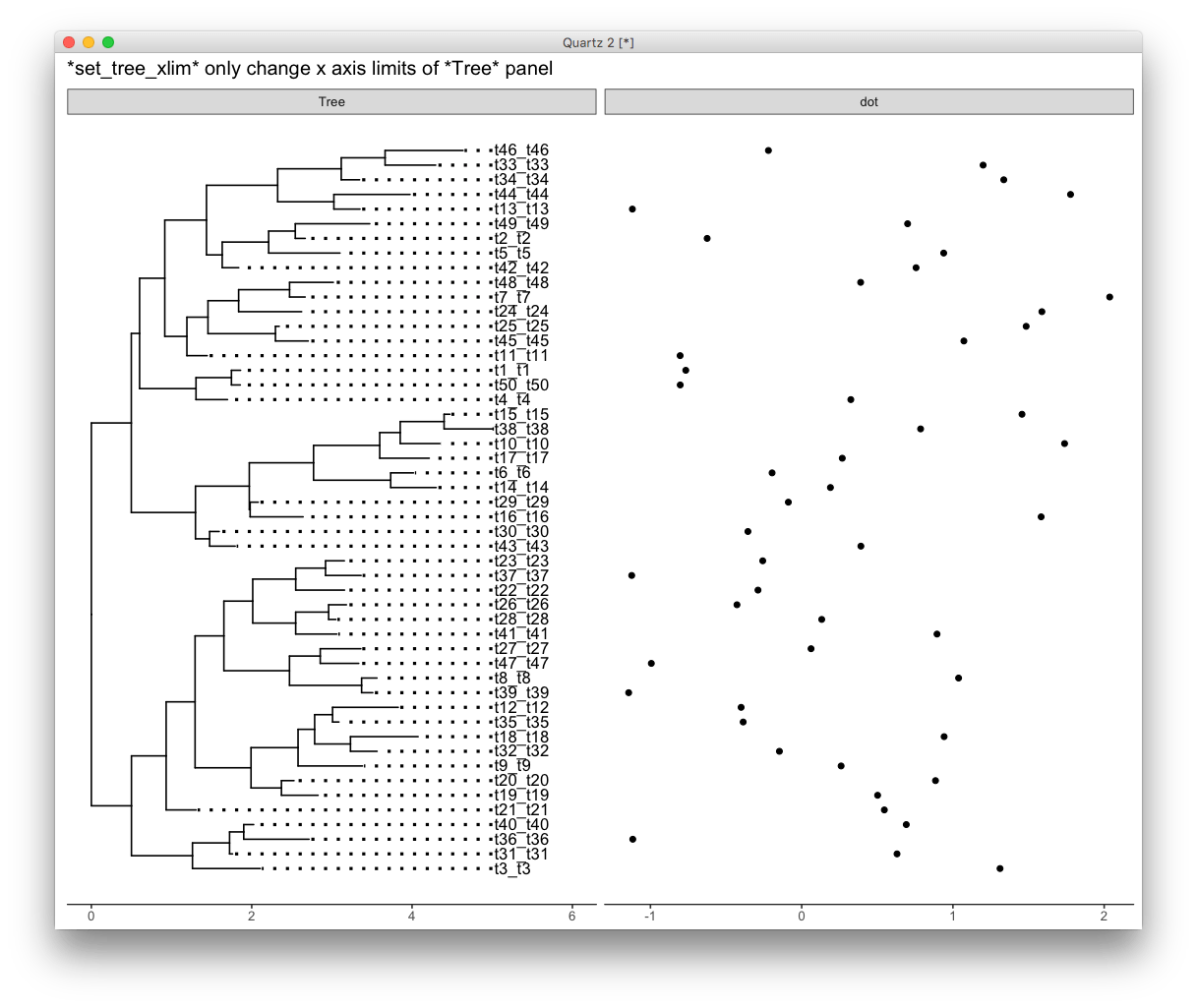# xlim_tree: set x axis limits for only Tree panel

October 30, 2016
By

Want to share your content on R-bloggers? click here if you have a blog, or here if you don't.

A ggtree user recently asked me the following question in google group:

I try to plot long tip labels in ggtree and usually adjust them using xlim(), however when creating a facet_plot xlim affects all plots and minimizes them.

Is it possible to work around this and only affect the tree and it’s tip labels leaving the other plots in facet_plot unaffected?

This is indeed a desire feature, as `ggplot2` can’t automatically adjust `xlim` for text since the units are in two different spaces (data and pixel).

Here is an example, the tip labels are truncated.

``````set.seed(2016-10-31)
tr =rtree(50)
tr\$tip.label = paste(tr\$tip.label, tr\$tip.label, sep="_")
p <- ggtree(tr) + geom_tiplab(align=TRUE) + theme_tree2()
d = data.frame(id = tr\$tip.label, v= rnorm(50))

facet_plot(p, geom=geom_point, data=d, mapping=aes(x=v), panel='dot') +
ggtitle("truncated tip labels")
``````If we only visualize the tree, it is easy to solve by using `xlim()` to allocate more space for the lables. But `xlim()` works for all panels. Combination of `facet_plot()` and `xlim()` will produce figure with many spaces.

``````facet_plot(p+xlim(NA, 6), geom=geom_point, data=d, mapping=aes(x=v), panel='dot') +
ggtitle("xlim applies to all panels")
``````To overcome this issue, `ggtree` provides `xlim_tree` to set `x` axis limits for only the `Tree` panel.

``````facet_plot(p+xlim_tree(6), geom=geom_point, data=d, mapping=aes(x=v), panel='dot') +
ggtitle('*xlim_tree* only change x axis limits of *Tree* panel')

# or using:
# facet_plot(p, geom=geom_point, data=d, mapping=aes(x=v), panel='dot') + xlim_tree(6)
``````## Citation

G Yu, DK Smith, H Zhu, Y Guan, TTY Lam*. ggtree: an R package for visualization and annotation of phylogenetic trees with their covariates and other associated data. Methods in Ecology and Evolution. `doi:10.1111/2041-210X.12628`.

R-bloggers.com offers daily e-mail updates about R news and tutorials about learning R and many other topics. Click here if you're looking to post or find an R/data-science job.
Want to share your content on R-bloggers? click here if you have a blog, or here if you don't.